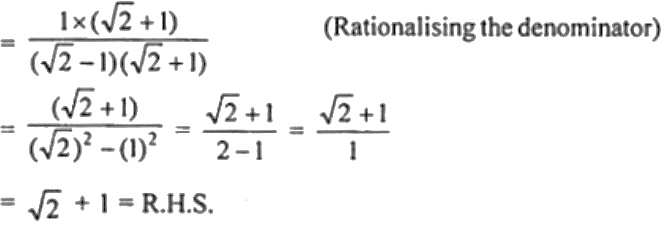Guru

# If sin θ + cos θ = √2 sin (90° – θ), show that cot θ = √2 + 1

• 0

This is an important question from the
Book- ML Aggarwal
Board- ICSE
Publication- Avichal
Chapter- Trigonometric Identities
Chapter number-18

If sin θ + cos θ = √2 sin (90° – θ), show that cot θ = √2 + 1

Trigonometric Identities, class 10th, chapter 18th, question no31 , ICSE board, ML aggarwal..

Share

1. Given, sin θ + cos θ = √2 sin (90° – θ)

sin θ + cos θ = √2 cos θ

On dividing by sin θ, we have

1 + cot θ = √2 cot θ

1 = √2 cot θ – cot θ

(√2 – 1) cot θ = 1

cot θ = 1/ (√2 – 1)Hence, cot θ = √2 + 1

• 0